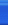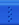# u-s-soldiersBlogJava首页新随笔联系聚合管理String str="abc efg ABC";

String regEx="a|f";   //表示a或f

Pattern p=Pattern.compile(regEx);

Matcher m=p.matcher(str);

boolean rs=m.find();

String regEx=".+\\\\(.+)\$";

String str="c:\\dir1\\dir2\\name.txt";

Pattern p=Pattern.compile(regEx);

Matcher m=p.matcher(str);

boolean rs=m.find();

for(int i=1;i<=m.groupCount();i++){

System.out.println(m.group(i));

}

String regEx="::";

Pattern p=Pattern.compile(regEx);

String[] r=p.split("xd::abc::cde");

String str="xd::abc::cde";

String[] r=str.split("::");

String regEx="a+"; //表示一个或多个a

Pattern p=Pattern.compile(regEx);

Matcher m=p.matcher("aaabbced a ccdeaa");

String s=m.replaceAll("A");

String s=m.replaceAll("");

\d 等於 [0-9] 数字
\D 等於 [^0-9] 非数字
\s 等於 [ \t\n\x0B\f\r] 空白字元
\S 等於 [^ \t\n\x0B\f\r] 非空白字元
\w 等於 [a-zA-Z_0-9] 数字或是英文字
\W 等於 [^a-zA-Z_0-9] 非数字与英文字

^ 表示每行的开头
\$ 表示每行的结尾
posted on 2008-05-03 14:44 u-s-soldiers 阅读(113) 评论(0)  编辑  收藏只有注册用户登录后才能发表评论。 网站导航: